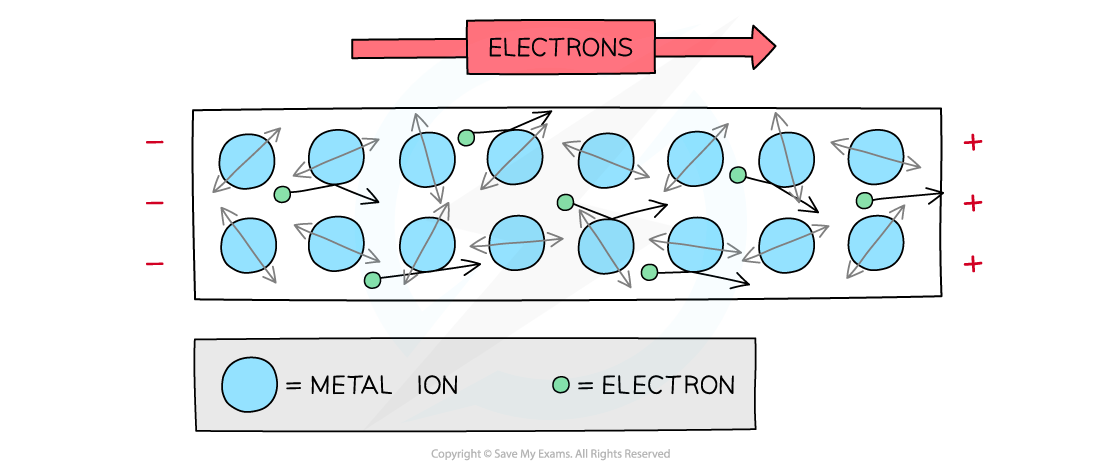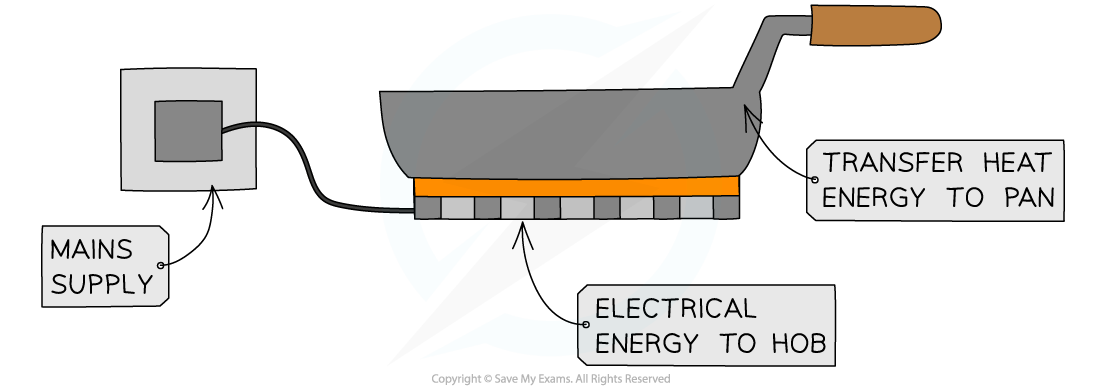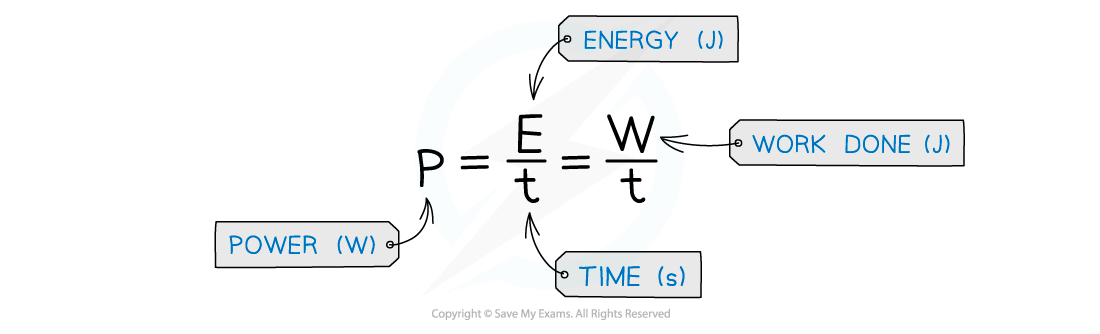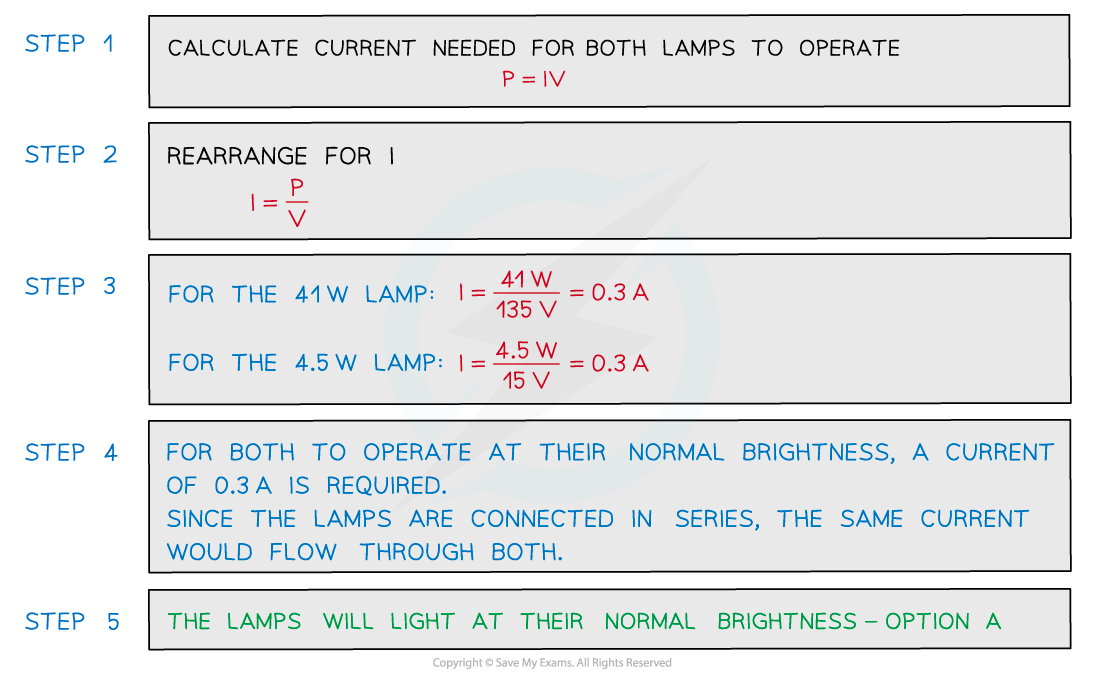# IB DP Physics: HL复习笔记5.2.8 Heating Effect of Current

### Heating Effect of Current

• When electricity passes through a component, such as a resistor, some of the electrical energy is turned into heat, therefore, increasing its temperature
• The heat that is produced will dissipate (spread out) into the environment via thermal conduction, convection and radiation
• When electricity passes through a component, there is energy transferred to heat
• This is due to collisions between:
• Electrons flowing in the conductor, and
• The lattice of atoms within the metal conductor
• Electricity, in metals, is caused by a flow of electrons
• This is called the current
• Metals are made up of a lattice of ions
• As the electrons pass through the metal lattice they collide with ions
• The ions resist the flow of the electronsAs electrons flow through the metal, they collide with ions, making them vibrate more

When the electrons collide, they lose some energy by giving it to the ions, which start to vibrate more

• As a result of this, the metal heats up
• This is used to an advantage to generate heat for appliances such as electric hobsThe heating effect of current can be used for many applications such as electric hobs

### Power Dissipation

• When an electrical current does work against electrical resistance:
• Electrical energy is dissipated as thermal energy in the surroundings
• The heat that is produced will dissipate via thermal conduction, convection and radiation
• The amount of heat produced depends on two factors:
• Current: The greater the current, the more heat that is produced
• Resistance: The higher the resistance, the more heat that is produced (for a given current)
• Note that reducing the resistance can cause the current to increase
• This could actually increase the amount of heat produced
• In mechanics, power P is defined as the rate of doing work
• The potential difference is the work done per unit charge
• Current is the rate of flow of charge
• Therefore, the electrical power is defined as the rate of change of work done:• The work done is the energy transferred so the power is the energy transferred per second in an electrical component
• The power dissipated (produced) by an electrical device can also be written as• Using Ohm's Law V = IR to rearrange for either V or I and substituting into the power equation, means power can be written in terms of resistance R• This means for a given resistor if the current or voltage doubles the power will be four times as great.
• Which equation to use will depend on whether the value of current or voltage has been given in the question
• Rearranging the energy and power equation, the energy can be written as:

E = VIt

• Where:
• E = energy transferred (J)
• V = voltage (V)
• I = current (A)
• t = time (s)

#### Worked Example

Two lamps are connected in series to a 150 V power supply.Which statement most accurately describes what happens?

A.     Both lamps light normally

B.     The 15 V lamp blows

C.     Only the 41 W lamp lightsD.     Both lamps light at less than their normal brightness